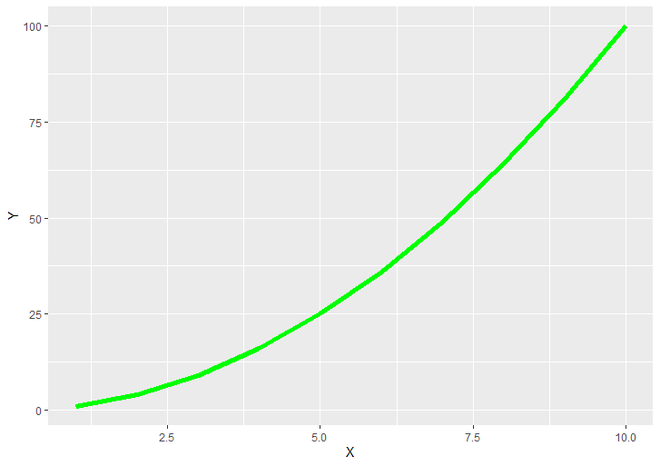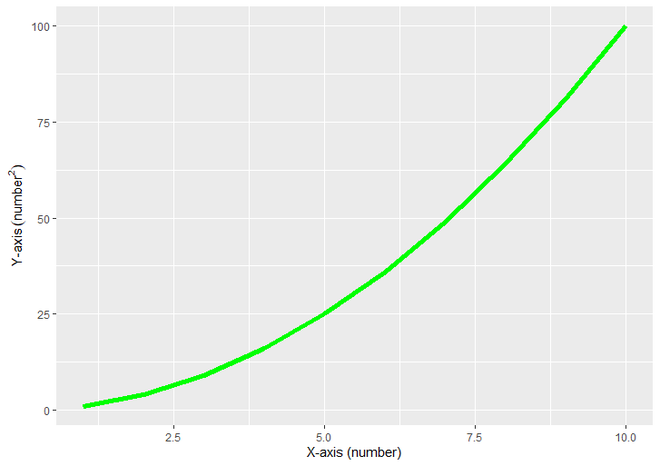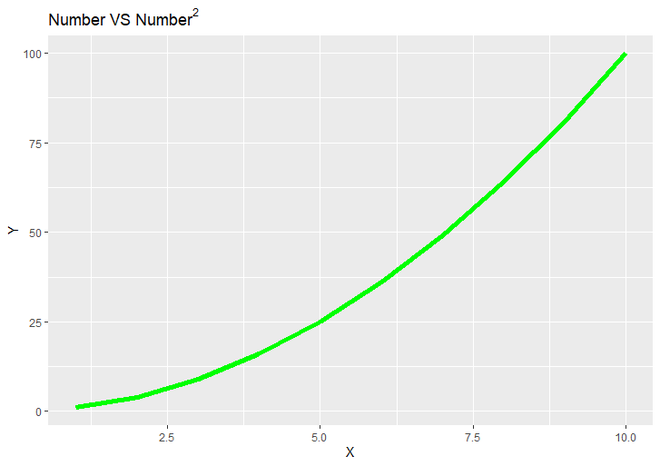# How to use superscript with ggplot2 in R?

• Last Updated : 17 Jun, 2021

In this article, we will see how to use superscript with ggplot2 in the R programming language. You can use Superscript anywhere in the plot where you want. The function will remain the same to use superscript values at all places. Here we will use superscript value at ggplot2 title and at the Label of Axis.

For that, the first ggplot2 package is loaded using the library() function.

Data in Use:

To create an R plot, we use ggplot() function and to make a line graph, add geom_line() function to ggplot() function. Let us first plot it regularly so that the difference is apparent.

Example:

## R

 `# Load Package``library``(``"ggplot2"``)`` ` `# Create a DataFrame ``DF <- ``data.frame``(X = ``c``(1, 2, 3, 4, 5, 6, 7,``                       ``8, 9, 10),          ``                  ` `                 ``Y = ``c``(1, 4, 9, 16, 25, 36, ``                       ``49, 64, 81, 100))`` ` `# Create a LineGraph``ggplot``(DF,``aes``(X, Y))+``  ``geom_line``(size = 2, color = ``"green"``)`

Output:Simple Line Graph

### Superscript the Label of Plot Axis

Here bquote() function  is uses to produce a superscript label.

Syntax : bquote(expr)

Parameter :

• expr : language object

bquote() For SuperScript :

bquote(‘string'(math superscript Notation))

For assigning the labels to X and Y axis, we will use xlab() and ylab() function to give labels to X and Y Axis respectively.

Syntax : xlab(“Label for X-Axis”)

Syntax : ylab(“Label for Y-Axis”)

Example:

## R

 `# Load ggplot2 Package``library``(``"ggplot2"``)`` ` `# Create a DataFrame For Plotting``DF <- ``data.frame``(X = ``c``(1, 2, 3, 4, 5, 6, ``                       ``7, 8, 9, 10),      ``                  ` `                 ``Y = ``c``(1, 4, 9, 16, 25, 36,``                       ``49, 64, 81, 100))`` ` `# Create ggplot2 Line Graph with ``# SuperScripted value of Label of ``# Y Axis.``ggplot``(DF,``aes``(X, Y))+``  ``geom_line``(size = 2, color = ``"green"``)+``  ``xlab``(``'X-axis (number)'``)+``  ``ylab``(``bquote``(``'Y-axis '``(number^2)))`

Output:ggplot2 plot with superscripted label of Y Axis

### Superscript the Plot Title

To add superscript as a title add bquote function with value inside ggtitle().

Syntax : ggtitle(“Title for Plot”)

Parameter :

• like xlab and ylab functions, we can give the title for plot directly using this function. Here we will bquote() function for writing Superscript value ( Number VS Number2 ) as a title of plot.

Return : Title to plot.

Example:

## R

 `# Load ggplot2 Package``library``(``"ggplot2"``)`` ` `# Create a DataFrame For Plotting``DF <- ``data.frame``(X = ``c``(1, 2, 3, 4, 5, 6, ``                       ``7, 8, 9, 10),     ``                  ` `                 ``Y = ``c``(1, 4, 9, 16, 25, 36, ``                       ``49, 64, 81, 100))`` ` `# Create ggplot2 Line Graph with SuperScripted``# value of Title of plot``ggplot``(DF,``aes``(X, Y))+``  ``geom_line``(size = 2, color = ``"green"``)+``  ``ggtitle``(``bquote``(``'Number VS'``~Number^2))`

Output:ggplot2 plot with superscripted Title

My Personal Notes arrow_drop_up